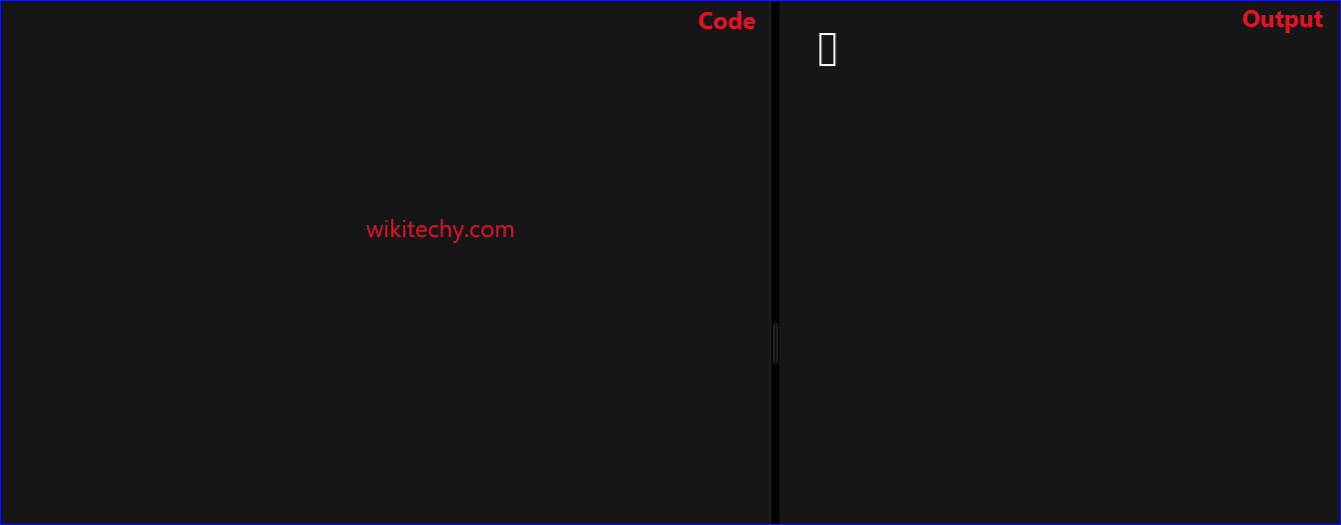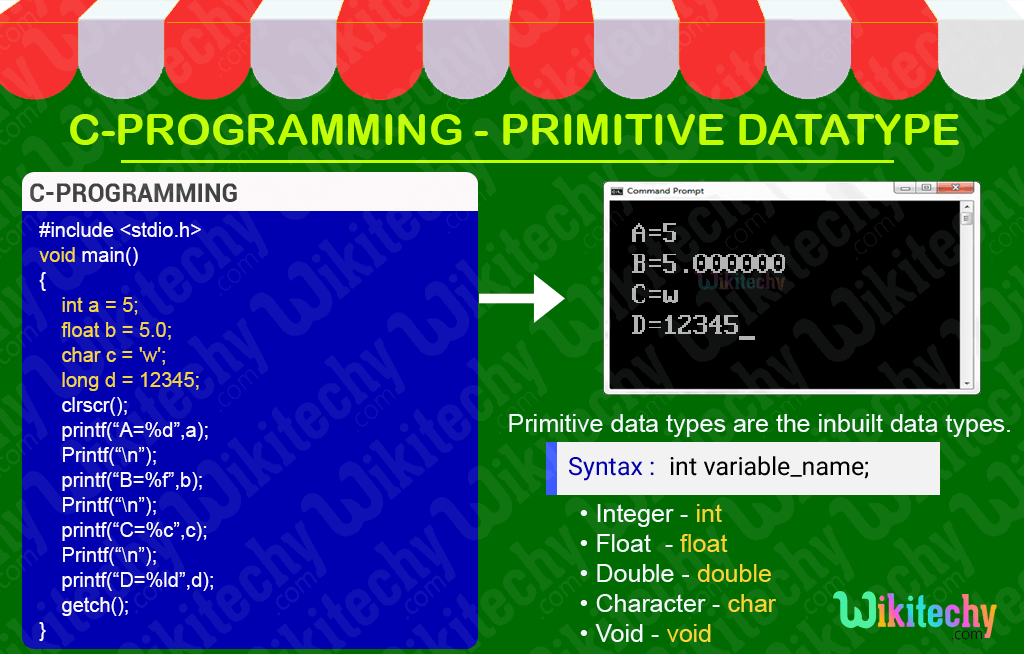# C - Primitive Data typesLearn C - C tutorial - Primitive data types - C examples - C programs

## C - Primitive Data types - Definition and Usage

• The primitive data types in c language are the inbuilt data types provided by the c language itself .
• Thus, all C compilers provide support for these data types. The Primitive Datatypes are as follows:
• Integer Data Type (int)
• Float data Type (float)
• Double Data Type (double)
• Character Data Type (char)
• Void Data Type (void)

Preprocessor directives in C## Integer Data Type (int) :

• Integer data type allows a variable to store numeric values (7,34,678,32…).
• int” keyword is used to refer integer data type.
• The storage size of int data type is 2 or 4 or 8 bytes.

## Float data Type (float) :

• Float data type declares a variable that can store numbers containing a decimal number (0.7 , 0.46, etc.) .
• “float” keyword is used to refer float data type.

## Character Data Type (char) :

• Character variables declared as char data type can only store one single character.
• “char” keyword is used to refer character data type.

## Void Data Type (void) :

• Void is an empty data type that has no value. Thus, we can say that it stores null.
• This can be used in functions and pointers.

Non Primitive data Types.

## C Code - Explanation :

1. Here in the c programming, void main() is declared as a parent function of all the functions in C. If we don't need any return value, we use return type 'void'.
2. In C programming, int a = 5 is specified as positive integer data type.
3. In C programming, float b = 5.0 is specified as positive float data type.
4.5. In C programming, char c = 'w' is specified as char data type.
6. In C programming, long d = 12345 is specified as long positive integer data type.
7. Here, printf() function is used to print the “integer, float, character, double values” onto the output screen where its format specifier is used to display the value of the variables.

## Sample Output - Programming Examples1. Here in this output A=5 specifies the integer data type.
2. Here in this output B=5.000000 specifies the float data type.
3. Here in this output C=w specifies the character data type.
4. Here in this output D=12345 specifies the long character data type.